# Introduction

We recently added visualizations based on Self-Organizing Maps (SOM) and Uniform Manifold Approximation and Projection (UMAP) for objects in the Mercator package. This addition relies on the core implementations in the kohonen and umap packages, respectively. The main challenge that we faced was that both of those implementations want to receive the raw data as inputs, while Mercator objects only store a distance matrix computed from the raw data. The purpose of this vignette is, first, to describe how we worked around this restriction, and second, to illustrate how to use these methods in the package.

# The Mercator Class

suppressMessages( suppressWarnings( library(Mercator) ) )

Next, we load a “fake” set of synthetic continuous data that comes with the Mercator package.

set.seed(36766)
data(fakedata)
ls()
##   "C"         "Cl4"       "Delta"     "J"         "N"         "P"
##   "SV"        "X"         "fakeclin"  "fakedata"  "filename"  "hclass"
##  "jacc.Vis"  "kk"        "mercury"   "my.binmat" "my.clust"  "my.data"
##  "neptune"   "pts"       "sokal.Vis" "tab"       "temp.Vis"  "venus"
dim(fakedata)
##  776 300
dim(fakeclin)
##  300   4

# UMAP

Let’s create a UMAP visualization directly from the raw data.

library(umap)
udirect <- umap(t(fakedata))
plot(udirect$layout, main="Original UMAP")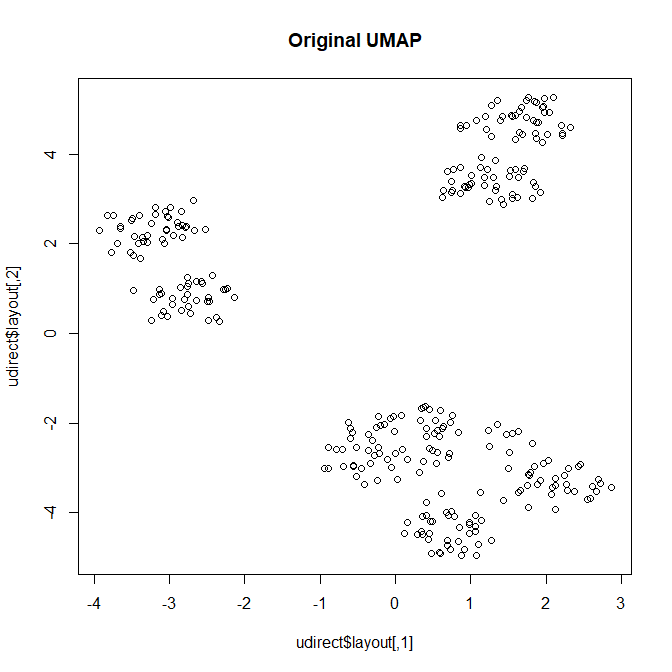We aren’t going to do anything fancy with labels or colors in this plot; we just want an idea of the main structure in the data. In particular, it looks like there are seven clusters, divided into one group three and two groups of two each. Next, we are going to create a Meractor object with a multi-dimensional scaling (MDS) visualization. Inspired by the first plot, we will arbitrarily assign seven groups. (This assignment uses the PAM algorithm internally.) mercury <- Mercator(dist(t(fakedata)), "euclid", "mds", 7) plot(mercury, main = "MDS")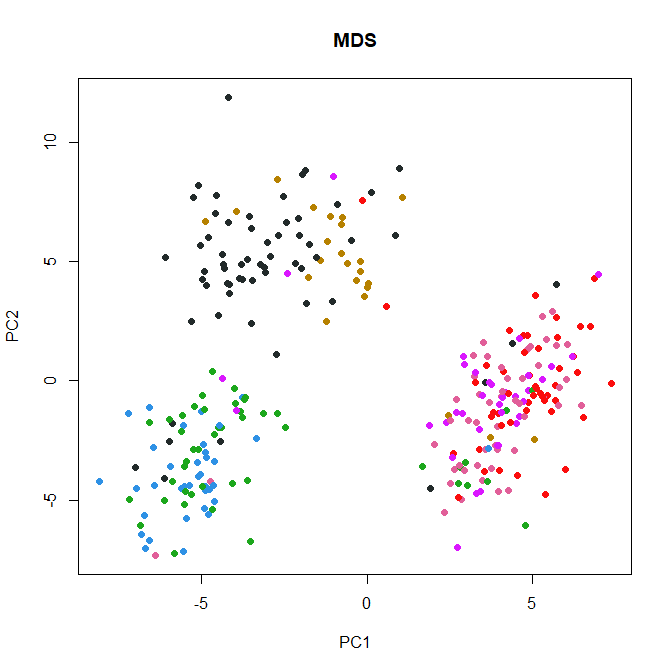Interestingly, here we see a possibly different number of groups. But that’s a separate issue, and we don’t want to distracted from our main thread. We want to confirm that the actual raw data is not contained in mercury, just the distance matrix. summary(mercury) ## An object of the 'Mercator' class, using the ' euclid ' metric, of size ##  300 300 ## Contains these visualizations: mds slotNames(mercury) ##  "metric" "distance" "view" "palette" "symbols" "clusters" mercury@metric ##  "euclid" mercury@palette ## NC1 NC2 NC3 NC4 NC5 NC6 NC7 NC8 ## "#2E91E5" "#E15F99" "#1CA71C" "#FB0D0D" "#DA16FF" "#222A2A" "#B68100" "#750D86" ## NC9 NC10 NC11 NC12 NC13 NC14 NC15 NC16 ## "#EB663B" "#511CFB" "#00A08B" "#FB00D1" "#FC0080" "#B2828D" "#6C7C32" "#778AAE" ## NC17 NC18 NC19 NC20 NC21 NC22 NC23 NC24 ## "#862A16" "#A777F1" "#620042" "#1616A7" "#DA60CA" "#6C4516" "#0D2A63" "#AF0038" mercury@symbols ##  16 15 17 18 10 7 11 9 table(mercury@clusters) ## ## 1 2 3 4 5 6 7 ## 36 50 46 48 36 61 23 class(mercury@view) ##  "list" names(mercury@view) ##  "mds" class(mercury@view[["hclust"]]) ##  "NULL" class(D <- mercury@distance) ##  "dist" Next, we add a “umap” visualization to this object. mercury <- addVisualization(mercury, "umap") plot(mercury, view = "umap", main="UMAP from distance matrix")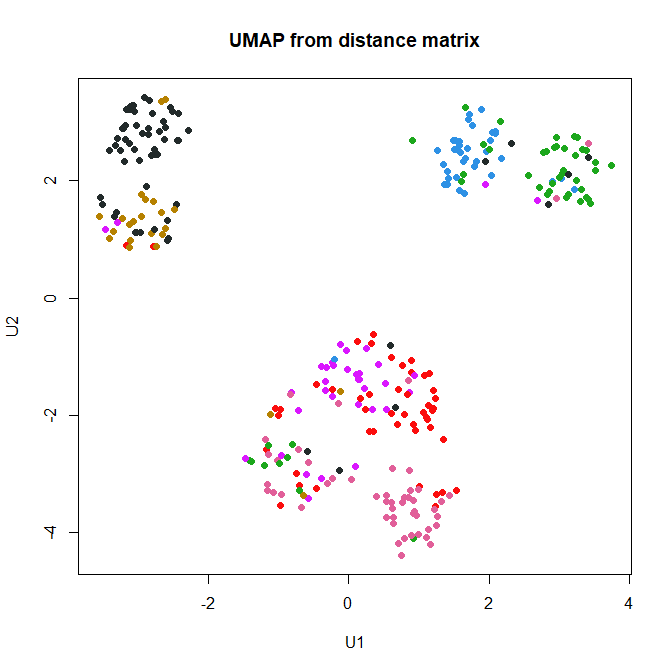Notice that the plot method knows that we want to see the internal “layout” component of the umap object. It also automatically assigns axis names that (subliminally?) remind us that we computed this visualization with UMAP. And it colors the points using the same values from the initial PAM clustering assignments. As an aside, we point out that the PAM clustering doesn’t really match the implicit UMAP clustering of the data. # Euclideanization We obviously constructed this plot just from the distance matrix, not from the raw data. It neverheless has a structure essentially the same as the original one. But how? Well, internally, we use this function: Mercator:::makeEuclidean ## function (D) ## { ## M <- as.matrix(D) ## X <- scale(t(scale(t(M^2), scale = FALSE)), scale = FALSE) ## E <- eigen(-X/2, symmetric = TRUE)$values
##     R <- sum(E > 1e-10)
##     cmdscale(D, k = R)
## }
## <bytecode: 0x0000000023c3c2b8>
## <environment: namespace:Mercator>

The first four lines of code inside this function are basically the algorithm used by the cmdscale function to compute the eigenvalues need to create its dimension reduction for visualization. The count of the number of nonzero eigenvalues (which defines R) is careful to stay away from zero. We do this to avoid round-off errors. If we miss by one or two eignevalues, than the final call to cmdscale will generate a confusing warning. That final line, by the way, creates an embedding into Euclidean space that should preserve almost all of the distances between pairs of points in the original data set.

To see that, let’s carry out that step explicitly.

X <- Mercator:::makeEuclidean(D)
dim(X)
##  300 299

Now we compute distances between pairs of points in this new embedding.

D2 <- dist(X)
delta <- as.matrix(D) - as.matrix(D2)
summary(as.vector(delta))
##       Min.    1st Qu.     Median       Mean    3rd Qu.       Max.
## -1.101e-13 -1.776e-14  0.000e+00 -1.496e-15  1.421e-14  1.066e-13

We see that the absolute errors between any pair of points in the two distance computations are less than $$10^{-12}$$. And that is the fundamental reason that we expect equivalent structures whether we apply UMAP to the original data or to the re-embedded data arising from the distance matrix.

# Self-Organizing Maps

The same approach works to compute self-organizing maps from a distance matrix. Here is the result using the data matrix.

mercury <- addVisualization(mercury, "som",
grid = kohonen::somgrid(6, 5, "hexagonal"))
plot(mercury, view = "som")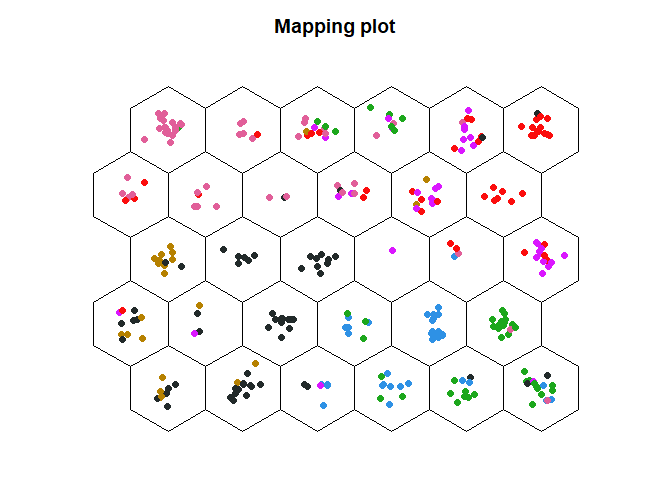And here is the corresponding result using the original data.

library(kohonen)
mysom <- som(t(fakedata), grid = somgrid(6, 5, "hexagonal"))
plot(mysom, type = "mapping")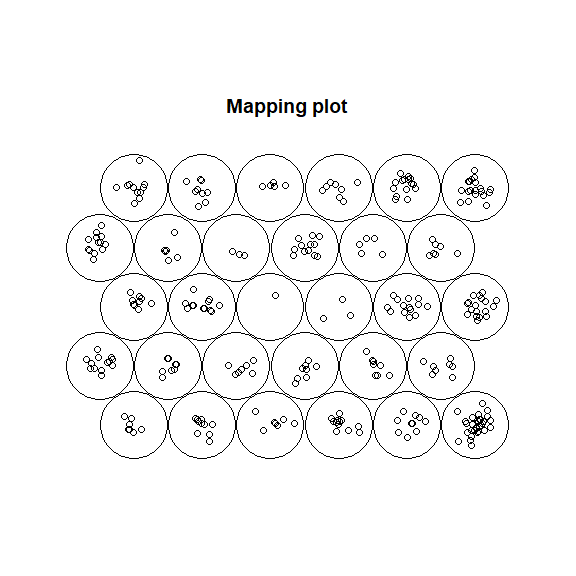This time, we will work a little harder to color the plot in the same way.

plot(mysom, type = "mapping", pchs=16, col=Mercator:::colv(mercury))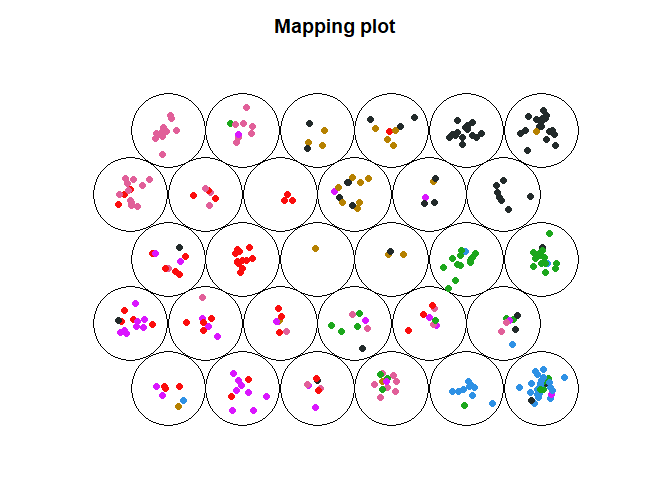SOM visualizations from a distance matrix.

## Warning

Qualitatively, most of the SOM plots should be similar. The fundamental exception, however, is the “codes” plot. This plot shows the patterns of intensities in each of the underlying dimensions (averaged over the assigned objects in each node). Because we have performed a multi-dimensional scaling analysis, we have changed the underlying coordinate system. Here are the corresponding plots.

plot(mysom, type = "codes", main="Original", shape = "straight")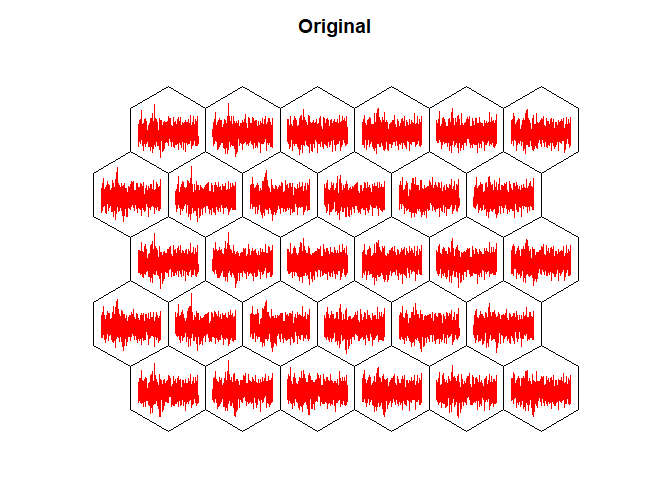SOM codes.

plot(mercury, view = "som", type="codes", main="After MDS")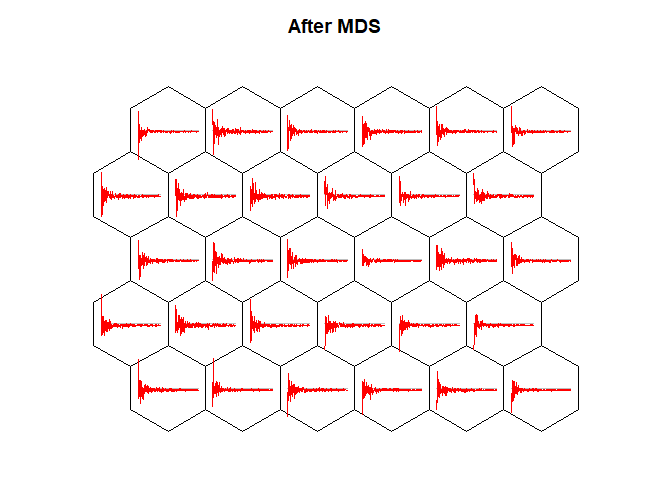SOM codes.

# Conclusions

As we have seen, when we start with a Euclidean distance matrix, we can get qualitatively equivalent results from the original data or from creating a “realization” of the distance matrix in a large Euclidean space. The major advantage, however, arises when we start with a completely different distance metric. In that case, we cannot apply SOM or UMAP at all. But we can still embed that distance matrix into a Eucldean space and then run SOM or UMAP.

# Appendix

This analaysis was performed in the following environment:

sessionInfo()
## R version 4.1.0 (2021-05-18)
## Platform: x86_64-w64-mingw32/x64 (64-bit)
## Running under: Windows 10 x64 (build 19041)
##
## Matrix products: default
##
## locale:
##  LC_COLLATE=C
##  LC_CTYPE=English_United States.1252
##  LC_MONETARY=English_United States.1252
##  LC_NUMERIC=C
##  LC_TIME=English_United States.1252
##
## attached base packages:
##  stats     graphics  grDevices utils     datasets  methods   base
##
## other attached packages:
##  kohonen_3.0.10       umap_0.2.7.0         Mercator_1.1.1
##  Thresher_1.1.3       PCDimension_1.1.11   ClassDiscovery_3.4.0
##  oompaBase_3.2.9      cluster_2.1.2
##
## loaded via a namespace (and not attached):
##   viridis_0.6.1        sass_0.4.0           jsonlite_1.7.2
##   viridisLite_0.4.0    bslib_0.2.5.1        assertthat_0.2.1
##  yaml_2.2.1           slam_0.1-48          pillar_1.6.1
##  lattice_0.20-44      glue_1.4.2           reticulate_1.20
##  digest_0.6.27        skmeans_0.2-13       colorspace_2.0-1
##  pkgconfig_2.0.3      purrr_0.3.4          cpm_2.3
##  scales_1.1.1         RSpectra_0.16-0      Rtsne_0.15
##  tibble_3.1.2         openssl_1.4.4        generics_0.1.0
##  ggplot2_3.3.4        movMF_0.2-5          ellipsis_0.3.2
##  nnet_7.3-16          magrittr_2.0.1       crayon_1.4.1
##  mclust_5.4.7         evaluate_0.14        fansi_0.5.0
##  MASS_7.3-54          changepoint_2.2.2    tools_4.1.0
##  lifecycle_1.0.0      stringr_1.4.0        kernlab_0.9-29
##  tidyselect_1.1.1     xfun_0.24